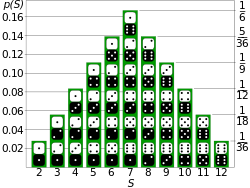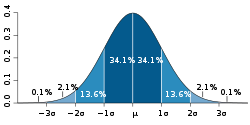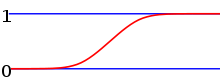# Probability distribution

﻿
Probability distribution

In probability theory, a probability mass, probability density, or probability distribution is a function that describes the probability of a random variable taking certain values.

For a more precise definition one needs to distinguish between discrete and continuous random variables. In the discrete case, one can easily assign a probability to each possible value: when throwing a die, each of the six values 1 to 6 has the probability 1/6. In contrast, when a random variable takes values from a continuum, probabilities are nonzero only if they refer to finite intervals: in quality control one might demand that the probability of a "500 g" package containing between 500 g and 510 g should be no less than 98%.Discrete probability distribution for the sum of two dice.Normal distribution, also called Gaussian or "bell curve", the most important continuous random distribution.

If total order is defined for the random variable, the cumulative distribution function gives the probability that the random variable is not larger than a given value; it is the integral of the non-cumulative distribution.

## Terminology

As probability theory is used in quite diverse applications, terminology is not uniform and sometimes confusing. The following terms are used for non-cumulative probability distribution functions:

• Probability mass, Probability mass function, p.m.f.: for discrete random variables.
• Categorical distribution: for discrete random variables with a finite set of values.
• Probability density, Probability density function, p.d.f: Most often reserved for continuous random variables.

The following terms are somewhat ambiguous as they can refer to non-cumulative or cumulative distributions, depending on authors' preferences:

• Probability distribution function: Continuous or discrete, non-cumulative or cumulative.
• Probability function: Even more ambiguous, can mean any of the above, or anything else.

Finally,

• Probability distribution: Either the same as probability distribution function. Or understood as something more fundamental underlying an actual mass or density function.

### Basic terms

• Mode: most frequently occurring value in a distribution
• Tail: region of least frequently occurring values in a distribution

## Discrete probability distributionThe probability mass function of a discrete probability distribution. The probabilities of the singletons {1}, {3}, and {7} are respectively 0.2, 0.5, 0.3. A set not containing any of these points has probability zero.The cdf of a discrete probability distribution, ...... of a continuous probability distribution, ...... of a distribution which has both a continuous part and a discrete part.

A discrete probability distribution shall be understood as a probability distribution characterized by a probability mass function. Thus, the distribution of a random variable X is discrete, and X is then called a discrete random variable, if

 ∑ Pr(X = u) = 1 u

as u runs through the set of all possible values of X. It follows that such a random variable can assume only a finite or countably infinite number of values.

In cases more frequently considered, this set of possible values is a topologically discrete set in the sense that all its points are isolated points. But there are discrete random variables for which this countable set is dense on the real line (for example, a distribution over rational numbers).

Among the most well-known discrete probability distributions that are used for statistical modeling are the Poisson distribution, the Bernoulli distribution, the binomial distribution, the geometric distribution, and the negative binomial distribution. In addition, the discrete uniform distribution is commonly used in computer programs that make equal-probability random selections between a number of choices.

### Cumulative density

Equivalently to the above, a discrete random variable can be defined as a random variable whose cumulative distribution function (cdf) increases only by jump discontinuities—that is, its cdf increases only where it "jumps" to a higher value, and is constant between those jumps. The points where jumps occur are precisely the values which the random variable may take. The number of such jumps may be finite or countably infinite. The set of locations of such jumps need not be topologically discrete; for example, the cdf might jump at each rational number.

### Delta-function representation

Consequently, a discrete probability distribution is often represented as a generalized probability density function involving Dirac delta functions, which substantially unifies the treatment of continuous and discrete distributions. This is especially useful when dealing with probability distributions involving both a continuous and a discrete part.

### Indicator-function representation

For a discrete random variable X, let u0, u1, ... be the values it can take with non-zero probability. Denote$\Omega_i=\{\omega: X(\omega)=u_i\},\, i=0, 1, 2, \dots$

These are disjoint sets, and by formula (1)$\Pr\left(\bigcup_i \Omega_i\right)=\sum_i \Pr(\Omega_i)=\sum_i\Pr(X=u_i)=1.$

It follows that the probability that X takes any value except for u0, u1, ... is zero, and thus one can write X as$X=\sum_i u_i 1_{\Omega_i}$

except on a set of probability zero, where 1A is the indicator function of A. This may serve as an alternative definition of discrete random variables.

## Continuous probability distribution

A continuous probability distribution shall be understood as a probability distribution that has a probability density function. Mathematicians also call such distribution absolutely continuous, since its cumulative distribution function is absolutely continuous with respect to the Lebesgue measure λ. If the distribution of X is continuous, then X is called a continuous random variable. There are many examples of continuous probability distributions: normal, uniform, chi-squared, and others.

Intuitively, a continuous random variable is the one which can take a continuous range of values — as opposed to a discrete distribution, where the set of possible values for the random variable is at most countable. While for a discrete distribution an event with probability zero is impossible (e.g. rolling 3½ on a standard die is impossible, and has probability zero), this is not so in the case of a continuous random variable. For example, if one measures the width of an oak leaf, the result of 3½ cm is possible, however it has probability zero because there are uncountably many other potential values even between 3 cm and 4 cm. Each of these individual outcomes has probability zero, yet the probability that the outcome will fall into the interval (3 cm, 4 cm) is nonzero. This apparent paradox is resolved by the fact that the probability that X attains some value within an infinite set, such as an interval, cannot be found by naively adding the probabilities for individual values. Formally, each value has an infinitesimally small probability, which statistically is equivalent to zero.

Formally, if X is a continuous random variable, then it has a probability density function ƒ(x), and therefore its probability to fall into a given interval, say [a, b] is given by the integral$\Pr[a\le X\le b] = \int_a^b f(x) \, dx$

In particular, the probability for X to take any single value a (that is aXa) is zero, because an integral with coinciding upper and lower limits is always equal to zero.

The definition states that a continuous probability distribution must possess a density, or equivalently, its cumulative distribution function be absolutely continuous. This requirement is stronger than simple continuity of the cdf, and there is a special class of distributions, singular distributions, which are neither continuous nor discrete nor their mixture. An example is given by the Cantor distribution. Such singular distributions however are never encountered in practice.

Note on terminology: some authors use the term"continuous distribution" to denote the distribution with continuous cdf. Thus, their definition includes both the (absolutely) continuous and singular distributions.

By one convention, a probability distribution$\,\mu$ is called continuous if its cumulative distribution function$F(x)=\mu(-\infty,x]$ is continuous and, therefore, the probability measure of singletons$\mu\{x\}\,=\,0$ for all$\,x$.

Another convention reserves the term continuous probability distribution for absolutely continuous distributions. These distributions can be characterized by a probability density function: a non-negative Lebesgue integrable function$\,f$ defined on the real numbers such that$F(x) = \mu(-\infty,x] = \int_{-\infty}^x f(t)\,dt.$

Discrete distributions and some continuous distributions (like the Cantor distribution) do not admit such a density.

### Probability distributions of real-valued random variables

Because a probability distribution Pr on the real line is determined by the probability of a real-valued random variable X being in a half-open interval (-∞, x], the probability distribution is completely characterized by its cumulative distribution function:$F(x) = \Pr \left[ X \le x \right] \qquad \forall x \in \mathbb{R}.$

### Terminology

The support of a distribution is the smallest closed interval/set whose complement has probability zero. It may be understood as the points or elements that are actual members of the distribution.

### Some properties

• The probability density function of the sum of two independent random variables is the convolution of each of their density functions.
• The probability density function of the difference of two independent random variables is the cross-correlation of their density functions.
• Probability distributions are not a vector space – they are not closed under linear combinations, as these do not preserve non-negativity or total integral 1 – but they are closed under convex combination, thus forming a convex subset of the space of functions (or measures).

## Random number generation

A frequent problem in statistical simulations (Monte Carlo method) is the generation of pseudo-random numbers that are distributed in a given way. Most algorithms are based on a pseudorandom number generator that produces numbers X that are uniformly distributed in the interval [0,1). These X are then transformed to some u(X) that satisfy a given distribution f(u).

## Kolmogorov definition

In the measure-theoretic formalization of probability theory, a random variable is defined as a measurable function X from a probability space$(\Omega, \mathcal{F}, \operatorname{P})$ to measurable space$(\mathcal{X},\mathcal{A})$. A probability distribution is the pushforward measure X*P = PX −1 on$(\mathcal{X},\mathcal{A})$.

## Applications

The concept of the probability distribution and the random variables which they describe underlies the mathematical discipline of probability theory, and the science of statistics. There is spread or variability in almost any value that can be measured in a population (e.g. height of people, durability of a metal, sales growth, traffic flow, etc.); almost all measurements are made with some intrinsic error; in physics many processes are described probabilistically, from the kinetic properties of gases to the quantum mechanical description of fundamental particles. For these and many other reasons, simple numbers are often inadequate for describing a quantity, while probability distributions are often more appropriate.

As a more specific example of an application, the cache language models and other statistical language models used in natural language processing to assign probabilities to the occurrence of particular words and word sequences do so by means of probability distributions.

## Common probability distributions

The following is a list of some of the most common probability distributions, grouped by the type of process that they are related to. For a more complete list, see list of probability distributions, which groups by the nature of the outcome being considered (discrete, continuous, multivariate, etc.)

Note also that all of the univariate distributions below are singly peaked; that is, it is assumed that the values cluster around a single point. In practice, actually observed quantities may cluster around multiple values. Such quantities can be modeled using a mixture distribution.

### Related to real-valued quantities that grow linearly (e.g. errors, offsets)

• Normal distribution (Gaussian distribution), for a single such quantity; the most common continuous distribution

### Related to real-valued quantities that are assumed to be uniformly distributed over a (possibly unknown) region

• Discrete uniform distribution, for a finite set of values (e.g. the outcome of a fair die)
• Continuous uniform distribution, for continuously distributed values

### Related to Bernoulli trials (yes/no events, with a given probability)

• Basic distributions:
• Related to sampling schemes over a finite population:
• Hypergeometric distribution, for the number of "positive occurrences" (e.g. successes, yes votes, etc.) given a fixed number of total occurrences, using sampling without replacement
• Beta-binomial distribution, for the number of "positive occurrences" (e.g. successes, yes votes, etc.) given a fixed number of total occurrences, sampling using a Polya urn scheme (in some sense, the "opposite" of sampling without replacement)

### Useful for hypothesis testing related to normally distributed outcomes

• Chi-squared distribution, the distribution of a sum of squared standard normal variables; useful e.g. for inference regarding the sample variance of normally distributed samples (see chi-squared test)
• Student's t distribution, the distribution of the ratio of a standard normal variable and the square root of a scaled chi squared variable; useful for inference regarding the mean of normally distributed samples with unknown variance (see Student's t-test)
• F-distribution, the distribution of the ratio of two scaled chi squared variables; useful e.g. for inferences that involve comparing variances or involving R-squared (the squared correlation coefficient)

### Useful as conjugate prior distributions in Bayesian inference

Wikimedia Foundation. 2010.

### Look at other dictionaries:

• Probability Distribution — A statistical function that describes all the possible values and likelihoods that a random variable can take within a given range. This range will be between the minimum and maximum statistically possible values, but where the possible value is… …   Investment dictionary

• probability distribution — The mathematical function describing the probability of different events, as described by values for a variable. A symmetrical distribution (one where to values are evenly distributed around the mean) is called a bell curve, a normal distribution …   Financial and business terms

• probability distribution — /prɒbəˈbɪləti dɪstrəˌbjuʃən/ (say probuh biluhtee distruh.byoohshuhn) noun a listing of several measurement categories, or classes, with the probability of the observation of each. See frequency distribution …   Australian English dictionary

• probability distribution — noun Date: 1920 probability function; also probability density function 2 …   New Collegiate Dictionary

• Probability distribution — Also called a probability function, a function that describes all the values that the random variable can take and the probability associated with each. The New York Times Financial Glossary …   Financial and business terms

• probability distribution — noun : probability function ; also : probability density function 2 …   Useful english dictionary

• probability distribution — noun A function of a discrete random variable yielding the probability that the variable will have a given value …   Wiktionary

• probability distribution — a mathematical function that assigns to each measurable event in a sample space the probability that the event will occur …   Medical dictionary

• probability distribution — Statistics. a distribution of all possible values of a random variable together with an indication of their probabilities. [1935 40] * * * …   Universalium

• probability distribution — probabil′ity distribu tion n. sta a distribution of all possible values of a random variable together with an indication of their probabilities …   From formal English to slang